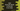# Java program to move all zeroes of an integer array to the end of the array### Java program to move all zero of an integer array to the end of the array:

In this tutorial, we will learn how to move all 0 of an integer array to the end of that array in Java. For example, for the array {1,0,2,0,3,0}, it will become {1,2,3,0,0,0}. The algorithm we are going to use is as below :

### Algorithm :

1. The array is given. Or we can get the inputs from the user.
2. Use one variable to indicate the current index of the array. Scan one by one element of the array.
3. If the value is not 0, insert this value to the current index . Also, increment the index value.
4. After all non zero values are inserted, append zero to all remaining positions of the array.
5. Print out the array.

### Java Program :

``````class Main {
public static void main(String args[]) {
//1
int[] inputArray = {3, 2, 1, 0, 4, 0, 0, 2, 7, 0, 50, 0, 6, 8, 9, 0, 9, 1, 0, 8, 5};

//2
int currentIndex = 0;

//3
for (int i = 0; i < inputArray.length; i++) {
//4
if (inputArray[i] != 0) {
inputArray[currentIndex] = inputArray[i];
currentIndex++;
}
}

//5
while (currentIndex < inputArray.length) {
inputArray[currentIndex] = 0;
currentIndex++;
}

//6
for (int i = 0; i < inputArray.length; i++) {
System.out.print(inputArray[i]+",");
}
}
}``````

### Explanation :

The commented numbers in the above program denote the step number below :

1. inputArray is the given array. It contains both zero and non-zero values.
2. Initialize one integer value currentIndex with value as 0.
3. Iterate through the array one by one element.
4. Check if the current element is non zero or not. If non-zero, insert the value to the position currentIndex. Increment the value of currentIndex.
5. After all items are scanned, insert 0 to all remaining position of the array.
6. Finally, print out the array to the user.

### Output :

``3,2,1,4,2,7,50,6,8,9,9,1,8,5,0,0,0,0,0,0,0,``

So, all zero values are moved to the last of the array.